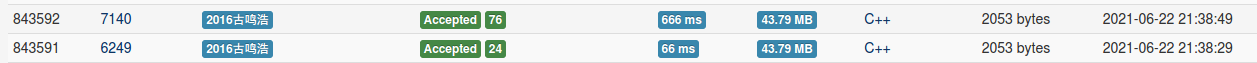# loj#3528. 「IOI2021」位移寄存器

①s=0,n,K<=2,Q<=20

②s=0

（与n无关的做法

③s=1

s=0的做法没有任何用处，考虑一开始的直接比较

# loj#3527. 「IOI2021」地牢游戏

（建图可以优化，把非[2^k,2^(k+1))的直接缩边，这样空间可以乘1/8

# loj#3524. 「IOI2021」钥匙

（因为每次连同一块的都是顶团，所以不会有连到的到不了自己这种情况

# loj#3523. 「IOI2021」分糖果

log^2的辣鸡做法

L=0,R=n-1感觉可以维护折线做，但是没什么扩展性

（把线段树分治去了就是一个log了

# 7152. 【2021.6.28 NOI模拟】bet

$$E_i(x^2)=\sum_x (p(ax+b)+(1-p)x)P_x$$，画一下得到

$$E_i(x^2)=(A\sum_x x^2P_x)+(B\sum_x xP_x)+C=AE_{i-1}(x^2)+BE_{i-1}(x)+C$$

# 7151. 【2021.6.28 NOI模拟】tennis

$$\large \sum_{l=1}^{n+m-1}\binom{l}{n}(1/2)^{l+1}(n+m-l)$$，m同理

$$\large F(S,n)=\sum_{l=n}^{n+m-1}\binom{l}{n}(1/2)^{l+1}$$，有

$$\large F(S,n)=[x^n]\frac{(1/2)^n(x+1)^n-(1/2)^S(x+1)^S}{1-x}$$

1/(1-x)相当于前缀和，拆开后推导一波得到

$$\large F(S+1,n+1)=F(S,n)-(1/2)^{S+1}\binom{S}{n+1}$$

$$\large F(S+1,n)=F(S,n)+(1/2)^{S+1}\binom{S}{n}$$

$$\large F(S-1,n-1)=F(S,n)+(1/2)^{S}\binom{S-1}{n}$$

$$\large F(S-1,n)=F(S,n)-(1/2)^{S}\binom{S-1}{n}$$

$$\large F(S,n)=[x^n]\frac{(1/2)^n(x+1)^n-(1/2)^S(x+1)^S}{1-x}$$

$$\large =\sum_{i=0}^{n} [x^i] (1/2)^n(x+1)^n-(1/2)^S(x+1)^S$$

$$\large =1-(1/2)^S\sum_{i=0}^{n} \binom{S}{i}$$

$$\large =1-(1/2)^S\binom{S}{n}$$

$$\large F(S,n)$$即可

# 7130. 【2021.6.18 NOI模拟】计算几何（cal）

$$ans=4\sum_{n=1}^N\sum_{m=1}^M ([(n,m)=1]+2[(n,m)=2])(N-n+1)(M-m+1)-2(N+M)$$

# 6249. 【NOI2019模拟2019.7.3】七星连珠

k进制fwt：实际就是对len维的0~k-1做循环卷积，乘上$$\omega^{ij}$$$$\omega^{-ij}/k$$，k=2就是(x+y,x-y)# 7136. 【2021.6.21NOI模拟】寻觅(help)

ls的神奇做法：分层，每层按n->1把pi以及前一个和后一个删掉，然后把pi染色

# CF1539

E：维护到i，i是左/右手合法的另一边的集合，每次判断i-1转手是否合法，加进去后再根据i的限制弹set头尾，如果一边不合法就全弹

F：只用考虑终点和中点的相对位置，硬点终点往左/往右，如往右就把<=的看作+1，>的看作-1，求往前/后的最大后缀/前缀和，线段树维护最后/2，往左同理

# 2021计蒜之道决赛 Day2T2 类斐波那契数据分析

$$Ans=\sum_{i=1}^{n} \sum_{j=1}^{i} [(i,j)=1] f_{(i,j+d)}$$

$$Ans=\sum_k h_k \sum_{k|i} \sum_{j=1}^{i} [(i,j)=1][j \equiv -d \mod k]$$

（前提：(k|i),(k,d)=1

$$\sum_{j=1}^{i} [x|(i,j)][j \equiv -d \mod k]$$

$$\sum_{a=0}^{i/k-1} [x|(i,ka-d)]$$

$$Ans=\sum_k h_k \sum_{k|i} [(k,d)=1]\varphi(i)/\varphi(k)$$，O(nlogn)解决

# 牛客挑战赛51

A：枚举b的约数

B：先补成团，然后最大连次大

C：二分，或者直接枚举长度算

D：矩乘，注意没有合法方案时为0

E：变成φ然后旧试题，或者https://loj.ac/p/2476，跑不动

F：枚举深度，直接prufer不会算重

G：维护线段树每个区间的所属，有多个取区间最小的，一操作直接打标记，如果往下走到了一个新的所属就不走，删除用set维护每个区间的标号，O(nlog^2)，没写

# 7130. 【2021.6.18 NOI模拟】计算几何（cal）

$$ans=4\sum_{n=1}^N\sum_{m=1}^M ([(n,m)=1]+2[(n,m)=2])(N-n+1)(M-m+1)-2(N+M)$$

# 6249. 【NOI2019模拟2019.7.3】七星连珠

k进制fwt：实际就是对len维的0~k-1做循环卷积，乘上$$\omega^{ij}$$$$\omega^{-ij}/k$$，k=2就是(x+y,x-y)# 7136. 【2021.6.21NOI模拟】寻觅(help)

ls的神奇做法：分层，每层按n->1把pi以及前一个和后一个删掉，然后把pi染色

# CF1539

E：维护到i，i是左/右手合法的另一边的集合，每次判断i-1转手是否合法，加进去后再根据i的限制弹set头尾，如果一边不合法就全弹

F：只用考虑终点和中点的相对位置，硬点终点往左/往右，如往右就把<=的看作+1，>的看作-1，求往前/后的最大后缀/前缀和，线段树维护最后/2，往左同理

# 2021计蒜之道决赛 Day2T2 类斐波那契数据分析

$$Ans=\sum_{i=1}^{n} \sum_{j=1}^{i} [(i,j)=1] f_{(i,j+d)}$$

$$Ans=\sum_k h_k \sum_{k|i} \sum_{j=1}^{i} [(i,j)=1][j \equiv -d \mod k]$$

（前提：(k|i),(k,d)=1

$$\sum_{j=1}^{i} [x|(i,j)][j \equiv -d \mod k]$$

$$\sum_{a=0}^{i/k-1} [x|(i,ka-d)]$$

$$Ans=\sum_k h_k \sum_{k|i} [(k,d)=1]\varphi(i)/\varphi(k)$$，O(nlogn)解决

# 牛客挑战赛51

A：枚举b的约数

B：先补成团，然后最大连次大

C：二分，或者直接枚举长度算

D：矩乘，注意没有合法方案时为0

E：变成φ然后旧试题，或者https://loj.ac/p/2476，跑不动

F：枚举深度，直接prufer不会算重

G：维护线段树每个区间的所属，有多个取区间最小的，一操作直接打标记，如果往下走到了一个新的所属就不走，删除用set维护每个区间的标号，O(nlog^2)，没写

# 7105. 【2021.6.5 NOI模拟】想不想吃炸鱼呀（genshin）

50分算出每个区间的答案，然后把区间连到sam上，再把sam按next连

# 7123. 【2021.6.15NOI模拟】尼特（nit）

（非题解做法

i<j的把组合数C(l,i)的计算次数写出，发现可以用$$A*\binom{l}{i}+B*\binom{l}{i}*(l-i)$$来表示，由眼睛得A,B然后直接算一行组合数的一半，时间复杂度O(n)

0 1 2 3 4 5 6
8 6 4

0 1 2 3 4 5 6 7
10 8 6 4

597的做法：生成函数硬推求$$(x+x^{-1}+m-2)^{s}$$，可以短多项式求幂或者接着硬推？

# CF1534

3道交互……

A：判断

B：只有把宽为1的减才优

C：ai->bi，每个环*2

D：正常做法：询问1，然后把奇/偶层询问

E：暴力做法：每个数顺序枚举次数，最优显然是a,a,a-2,a-2...这样排，然后贪心判断n^3

F1：不想写tarjan，所以取了每列的最上段然后根据连通块连起来再求，最后直接去世

F2：在F1的基础上扩展，定义第j列的(a[j],j)为关键点，显然每个关键点都要操作，如果一个关键点能到达另一个关键点，就把被到达的删掉，把剩下的按原顺序重标号（如果一个块里标号不连续一定会被中间的删掉）

G：观察发现对于一个点(a,b)，一定是在斜线y=-x+(a+b)上操作最优

H：先求dp值，设f[i]表示在i的子树内确定一个端点的次数，求f时可以同时维护操作方案

# arc122

A：注意是没有相邻的，左右乘fib

B：化简得到Σ|x-Ai/2|+常数，取中位数

C：辣鸡构造：写成fib的和，维护x和y的已知位等于n的前若干位，然后用大的加到小的上来位移两位，这样x和y都要加上1的个数，所以次数是86+86

D：建trie往下找，找到左右有奇的就暴力取min（B控制），每部分再取max（答案）

E：正着不好搞，反着每次找一个能删掉的，对ai取gcd即可不超lcm

*F：

# arc121

A：离谱的做法：根据点的总矩形和每个点的位置求出max，边界-角计算框上的点数量，如果数量=1则第二大会小于max，找到那一对点，分别把两个删掉求剩余的max

B：全偶为0，否则有两个奇，显然要么是两种最近的匹配，要么和第三种各取min相加

C：从后往前每次把[i-1,i]中的某个丢到后面排序，这样最后会剩下一个，这个能移就直接移，否则可以通过操作a,a来把a变成2，然后对前三个轮换变成123

D：奇怪的做法：枚举前后缀选0，加上从外往内选，跨过0就选0，非常离谱但过了

E：容斥，每个点向祖先连就乘-1，设f[i,j]表示子树i内剩j个未连，子树背包

F：观察发现，不合法的充要条件是每个and块里有0，因为虽然可以用当前的1消掉其他的0，但最后总会剩下一个有0的块，设f[i,0/1]表示i子树内连通块的and为0/1的答案，转移

# 7099. 【2021.6.1 NOI模拟】传统游戏（nim）

polya容斥，枚举排列乘上(-1)^(逆序对个数)，也等于偶环个数，因为可以把两个标号交换（行列同时换，不变）做到重标号

A：

B：

C：

D：

E：

*F：

*G：

*H：

# 7095. 【2021.5.28 NOI模拟】异或矩阵(matrix)

posted @ 2021-06-02 15:34  gmh77  阅读(225)  评论(0编辑  收藏  举报